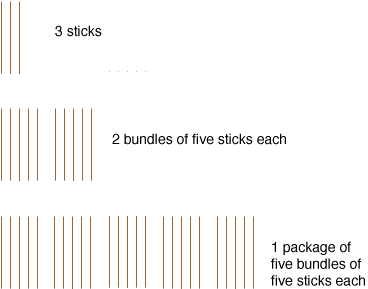Quandaries and Queries My sister is home schooled and we are trying to convert from base 10 to base 5. We are trying to teach and we have never had this in school. If we could have some examples of some problems and how to do them we would appreciate this. Thank you, Julie Hi Julie, Let me try an example. I am going to look at the number thirty-eight and I want to imagine that I have thirty-eight sticks. If I am thinking of base 10 ten I gather the sticks together into bundles of size ten sticks. I get three bundles of ten sticks each and then eight more sticks. In base ten then we write thirty-eight as 38. The 3 is the number of tens and the 8 is the number of units left. Now suppose that I want to write thirty-eight in base five. I first collect the sticks into bundles of five sticks each. I get seven bundles of five sticks each and 3 more sticks. Now I look at the seven bundles and gather then together into packages of five bundles each. I get one package of five bundles and 2 bundles remaining.Thus thirty-five, written in base five is 1235. The 1 is the number of twenty-fives, the 2 is the number of fives and the 3 is the number of units remaining. Arithmetically what I did was  38/5 = 7 with a remainder of 3  7/5 = 1 with a remainder of 2  1/5 = 0 with a remainder of 1 Reading the remainders from bottom to top I get 1235. What about one hundred and thirty-six?  136/5 = 27 with a remainder of 1 27/5 = 5 with a remainder of 2 5/5 = 1 with a remainder of 0 1/5 = 0 with a remainder of 1 136 = 10215 Penny